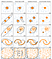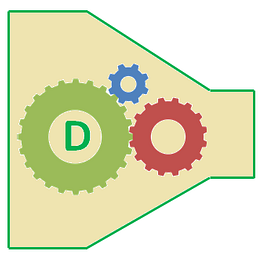# What’s the difference between novelty detection and outlier detection?

In novelty detection, you have a dataset that contains only good data and you are trying to check if new observations are similar to the the good data. In other words, our goal is to check if new observations are outliers.

In outlier detection, you dataset may already have outliers and your goal is to identify such outliers.

Both novelty detection and outlier detection are used to detect anomalies.

Outlier detection is an unsupervised anomaly detection algorithm.

Novelty detection is a semi-supervised anomaly detection algorithm.Outlier detection methods available in scikit-learn (LOF does not have a decision boundary as it does not have a predict method when used as an outlier detection algorithm)

# When would K-means clustering fail to give good results?

• Data points contain outliers
• Data points in non-convex/non-round shapes
• Data points with different densities

# What would be the best number of clusters for the following dendrogram?

You select the y that has the maximum range that does not change the cluster assignment.

# Given a dataset, what could change the shape of a dendrogram?

• Proximity function used
• Number of features used
• Number of data points used (sampling)

# How can Clustering (Unsupervised Learning) be used to improve the accuracy of Linear Regression model (Supervised Learning)?

• A clustering algorithm groups data points into n distinct groups. If these clusters are far apart and there are a lot of data, it may be a good idea to try creating a different regression models for different groups.
• Cluster information could be used as variables in the regression model as well. For example, cluster centroid value, cluster id, and cluster size could be utilized as three additional variables to the model.

# Data Preparation Framework for Machine Learning

• Remove duplicates from pandas dataframes [ref]
• Remove outliers from your dataset [ref]
• Data Sampling methods [ref]

• Feature selection [ref, ref]
• Polynomial features [ref]
• Label encoder vs. one-hot encoder [ref, ref]

• Data scaling [ref]
• Changing data distribution [ref]
• Imputation [ref]

• Dimensionality reduction [ref]

• Row consolidation

# How to Mitigate Bias Variance in Deep Learning Models?

In deep learning, you don’t have the bias-variance trade off as DL models allow you to reduce both!

A key observation is that in traditional ML, the performance reaches a pleatau after certain amount training data whereas in DL, performance improves with more training data.

# What is the difference between Type I and Type II errors?

• Type I error — False positive (e.g. telling a man he is pregnant)
• Type II error — False negative (e.g. telling a pregnant woman that she is not carrying a baby)

In Hypothesis testing, Type I error means we reject the Null hypothesis when the null is actually true. Type II error means we fail to reject the null when the null is not true.

• These two errors are inversely proportional.
• Type I error is represented by alpha which is the p-value below which we reject the null hypothesis. If p-value is 0.05, …

# How is KNN different from k-Means?

• Both algorithms coincidentally have the same k letter, but they are very different.
• KNN — K nearest neighbor — supervised algorithm (classification/regression), k-Means — unsupervised algorithm (clustering)
• Both use distance measurements but KNN needs labeled data whereas k-Means requires no data only a threshold on how many clusters we want to group the data into
• In KNN, each unlabeled data point is marked with the majority label of the k nearest data points. In k-Means, data points are grouped to into their nearest centroids.

# What is human-level performance?

This measures how well a human performs on a given classification task. For example, in a name entity recognition problem, if a human on average makes 15 errors out of 100 entities, the human level performance is 15%.

How do we measure human-level performance?

You need to go through manual labeling to measure this.

Get a group of people to label a stratified sample and then measure the average error.

This is also the Bayes Error.

This is the lowest error one can achieve from a ML model.

The difference between the Bayes Error and training error is called the…## Digestize

#probability #statistics #ML #DL #coding #security #building #digest